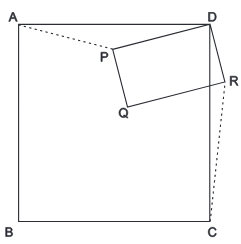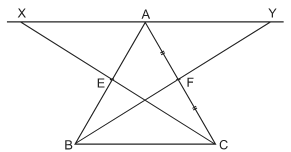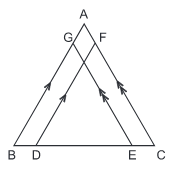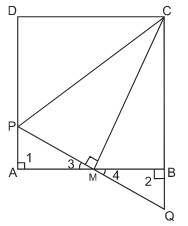# HOTS Questions: Triangles Notes | Study Mathematics (Maths) Class 9 - Class 9

## Class 9: HOTS Questions: Triangles Notes | Study Mathematics (Maths) Class 9 - Class 9

The document HOTS Questions: Triangles Notes | Study Mathematics (Maths) Class 9 - Class 9 is a part of the Class 9 Course Mathematics (Maths) Class 9.
All you need of Class 9 at this link: Class 9

Question 1. ABCD is a square. P is any point inside it, such that ΔPQR is another square. Prove that AP = CRHint: Join AP and CR.
In ΔADP and ΔCDR, we have :
AD = CD                      [sides of a square]
∠ADP = ∠CDR                     [each = 90° - ∠PDC]
DP = DR                    [side of a square]
⇒ ΔADP ≌ ΔCDR                     [SAS congruence]
⇒ AP = CR                     [C.P.C.T]

Question 2. E and F are the midpoints of sides AB, AC of ΔABC. CE and BF are produced to X and Y respectively, such that EX = CE and FY = BF. AX and AY are joined. Find in your figure, a triangle congruent to ΔAEX and demonstrate the congruency. Show that XAY is a straight line.Hint: Prove ΔAEX ≌ ΔBEC                    [By SAS congruency]
⇒ ∠ XAE = ∠ CBE                    [c.p.c.t.]
⇒ ∠XAB = ∠CBA
But they form a pair of co-interior angles.
⇒ XA || BC                    ...(1)
Similarly, ΔAFY ≌ ΔCFB
⇒ AY || BC                    ...(2)
from (1) and (2) XAY is a st. line.

Question 3. In the adjacent figure, BA || DF and CA || EG. If BD = EC then prove that BG = DF and EG = CF.Hint: In ΔGBE and ΔFDC ∠ABC = ∠FDE and ∠DED = ∠ACB
also BE = DC
∴ ΔGBE ≌ ΔFDC                    [ASA congruency]
⇒ BG = DF and EG = CF

Question 4. ABCD is a square. M is the midpoint of AB and PQ ⊥ CM meets AD at P. CB produced meet at Q. Prove that (i) PA = BQ and (ii) CP = AB + PAHint: Prove, ΔAMP ≌ ΔBMQ                    [ASA cong.]
⇒ MP = MQ and PA = QB                    [c.p.c.t.]
⇒ PA = BQ
Again, prove, ∆CMP ≌ ΔCMQ                    [SAS cong.]
⇒ CP = CQ                     [c.p.c.t.]
⇒ CP = CB + BQ = AB + PA

The document HOTS Questions: Triangles Notes | Study Mathematics (Maths) Class 9 - Class 9 is a part of the Class 9 Course Mathematics (Maths) Class 9.
All you need of Class 9 at this link: Class 9Use Code STAYHOME200 and get INR 200 additional OFF

## Mathematics (Maths) Class 9

73 videos|352 docs|109 tests

Track your progress, build streaks, highlight & save important lessons and more!

,

,

,

,

,

,

,

,

,

,

,

,

,

,

,

,

,

,

,

,

,

;